+
Common Core: S.MD.2

# Expected Value

##### Rating:
(10)
• (2)
• (2)
• (1)
• (1)
• (4)
Author: Sophia Tutorial
##### Description:

Calculate the expected value of a given situation.

(more)
Tutorial

what's covered
This tutorial will discuss determining the expected value. Our discussion breaks down as follows:

1. Expected Value of a Probability Distribution
1. Expected Value of a Spinner
2. Expected Value of a Roulette Wheel

## 1. Expected Value of a Probability Distribution

Expected value is the long-term average taken by the outcomes from a chance experiment. It can sometimes be a confusing term, so we can also use the term mean or average of a probability distribution.

The expected value is found by adding the products of each individual outcome and its probability.

formula

Expected Value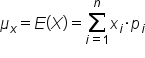hint
Notationally, we can write the expected value of a probability distribution for x as μx (mu meaning mean) or E(X) (E meaning expected value). Both of these are accepted notations, though typically, μ will be used.

term to know

Expected Value
The long-term average value taken by the outcomes from a chance experiment. It does not need to be one of the possible outcomes.

1a. Expected Value of a Spinner
The spinner below has three 1's, a 2, two 3's, and two 4's.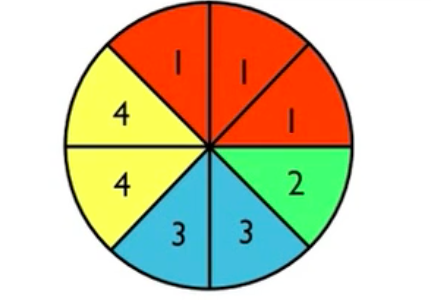The probability distribution is shown here. The outcomes are 1, 2, 3, and 4, and their probabilities are 3/8, 1/8, 2/8, and 2/8, respectively.

 Number Probability 1 2 3 4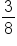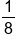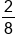When trying to determine an expected value (what is expected to happen), this expected value will be discussed as a long-term average value that the spinner might produce.

think about it
What do you think would happen if someone spun the spinner a number of times? Suppose this spinner was spun eight times and it went according to the expectations.

If spun eight times, the expectation would be for it to fall on each sector once. It doesn't have to happen that way, but it seems like that would be the expectation.

So if the spinner was spun eight times, we would expect three 1's, one 2, two 3's, and two 4's to be obtained. The law of large numbers says that the longer this is done (in the long term), this is exactly what will happen. About three out of every eight times, you'll get a 1. About one out of every eight times, you'll get a 2, etc. Therefore, you're going for the long-term average. Now, if that's the case, the mean is just going to be the average value from those eight spins: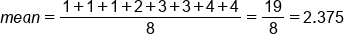The average value from those eight spins is 2.375. However, you can also rewrite that fraction by grouping the 1's, the 2's, the 3's, and the 4's. The 1 appeared three times, the 2 appeared one time, the 3 appeared twice, and the 4 appeared twice.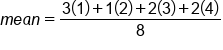You can continue breaking this down by having each addend individually divided by 8 and then added. So now it might look like this: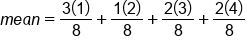Now, some of these look familiar. In fact, these fractions can be rewritten a different way. All of these fractions can be rewritten with the item in the parentheses pulled out in front of the remaining fraction, like this: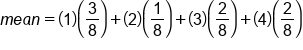These numbers are looking quite familiar, aren't they? It seems like these were the numbers from the probability distribution, where these numbers-- 1, 2, 3, and 4--were the potential outcomes, the values that will be referred to as "x". The fractions--the 3/8, 1/8, 2/8, and 2/8--were their probabilities.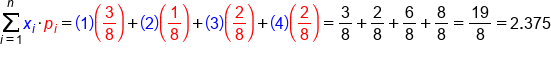This is how expected value will be calculated: the sum of each outcome times its individual probability.

The expected value, also called the mean of a probability distribution, is found by adding the products of each individual outcome and its probability. In this case, 2.375 was the result.

It is not a possibility on the spinner, nor is it an integer. Since the focus is about the long-term average, it doesn't actually need to be an integer. It doesn't need to be possible, either.

1b. Expected Value of a Roulette Wheel
Consider the roulette wheel below. There are 38 sectors: 18 red, 18 black, and 2 green. All the sectors are equally likely.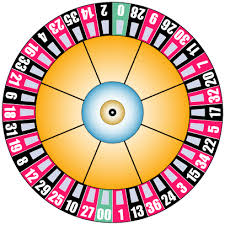What is the mean of a distribution showing the payouts and probabilities from betting red on a roulette wheel? If you bet \$1 and you win, you win \$1. If you don't win, you lose the dollar that you bet.

 Winnings Probability -1 1 20/38 18/38

There are 18 red sectors on a roulette wheel. There are 20, therefore, that aren't red, out of the 38. Find the expected value for a play on the roulette wheel.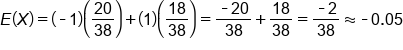One outcome is that you lose a dollar 20 out of 38 times. The other outcome is that you gain a dollar 18 out of 38 times. When you solve it, you end up with negative 2/38, which is about negative 0.05.

What this means is that over the long-term, you lose about a nickel every time you place a \$1 bet on a roulette wheel. Because it's negative, that means you will be losing money over the long-term.

summary
The expected value, also called the mean, of a probability distribution is taken by multiplying each probability times its outcome, then adding each of those products. It doesn't have to be an integer or a possible value.

Good luck!

Source: Adapted from Sophia tutorial by Jonathan Osters.

Terms to Know
Expected Value

The long-term average value taken by the outcomes from a chance experiment. It does not need to be one of the possible outcomes.

Formulas to Know
Expected ValueRating Header